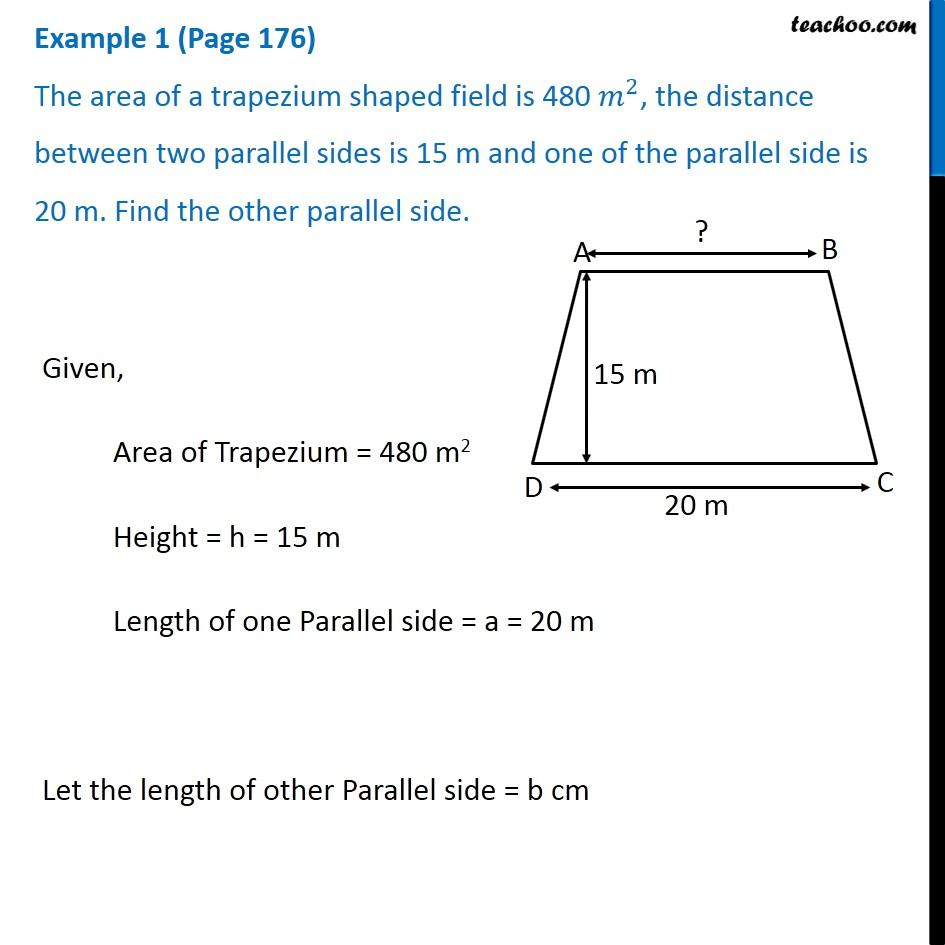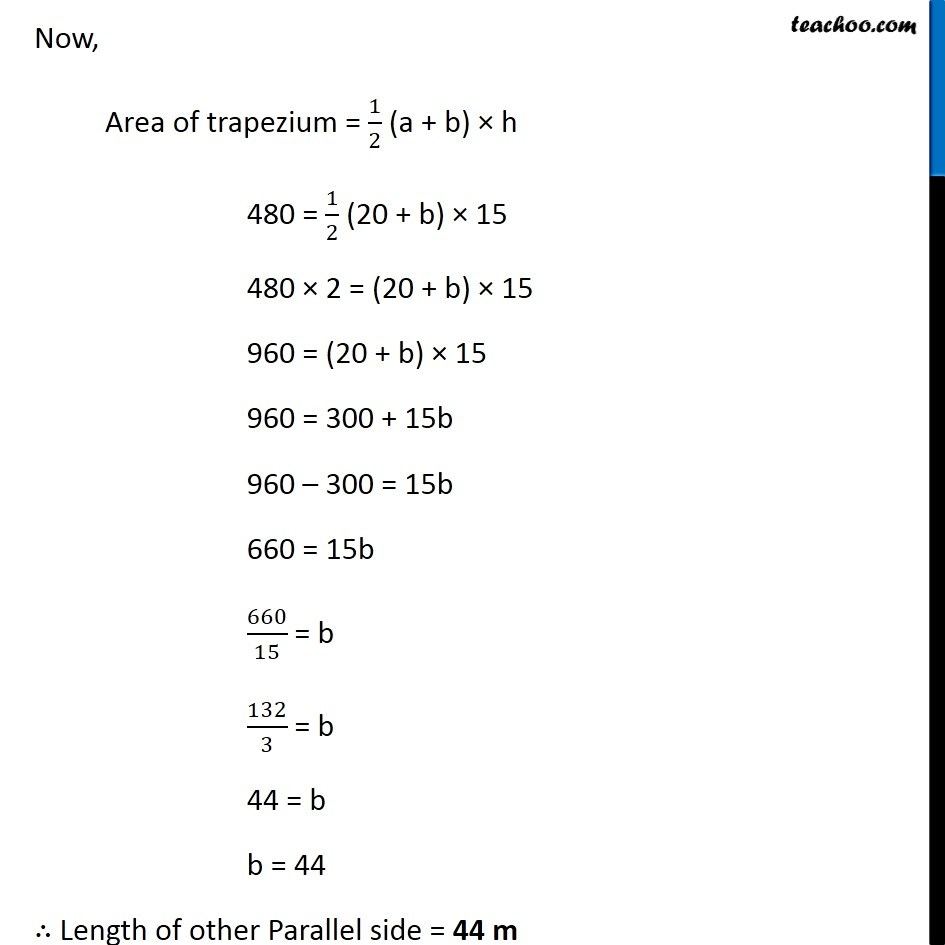Examples

Chapter 9 Class 8 Mensuration
Serial order wiseLearn in your speed, with individual attention - Teachoo Maths 1-on-1 Class

### Transcript

Example 1 (Page 176) The area of a trapezium shaped field is 480 𝑚^2, the distance between two parallel sides is 15 m and one of the parallel side is 20 m. Find the other parallel side. Given, Area of Trapezium = 480 m2 Height = h = 15 m Length of one Parallel side = a = 20 m Let the length of other Parallel side = b cm Now, Area of trapezium = 1/2 (a + b) × h 480 = 1/2 (20 + b) × 15 480 × 2 = (20 + b) × 15 960 = (20 + b) × 15 960 = 300 + 15b 960 – 300 = 15b 660 = 15b 660/15 = b 132/3 = b 44 = b b = 44 ∴ Length of other Parallel side = 44 m Example 2 (Page 177) The area of a rhombus is 240 〖𝑐𝑚〗^2 and one of the diagonals is 16 cm. Find the other diagonal. Given, Area of rhombus = 240 cm2 Length of one diagonal = d1 = 16 cm Let length of other diagonal = d2 = x cm. Now, Area of rhombus = 1/2 × d1 × d2 240 = 1/2 × 16 × x 240/8 = x 30 = x x = 30 cm ∴ The length of other diagonal = 30 cm.